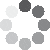Enter parameters
Enter parameters
Total Run:
ft
• inches (in)
• feet (ft)
• yards (yd)
• centimeters (cm)
• meters (m)
Total Rise:
ft
• inches (in)
• feet (ft)
• yards (yd)
• centimeters (cm)
• meters (m)
Number Of Steps:
Stringer Width:
in
• inches (in)
• feet (ft)
• yards (yd)
• centimeters (cm)
• meters (m)
Stringer Thickness:
in
• inches (in)
• feet (ft)
• yards (yd)
• centimeters (cm)
• meters (m)
Stair Width:
ft
• inches (in)
• feet (ft)
• yards (yd)
• centimeters (cm)
• meters (m)
in
• inches (in)
• feet (ft)
• yards (yd)
• centimeters (cm)
• meters (m)
in
• inches (in)
• feet (ft)
• yards (yd)
• centimeters (cm)
• meters (m)
Riser Thickness:
in
• inches (in)
• feet (ft)
• yards (yd)
• centimeters (cm)
• meters (m)
Stringer Mount Type: ?
Standard
Flush
Reset
Result
Run:
0
in
• inches (in)
• feet (ft)
• yards (yd)
• centimeters (cm)
• meters (m)
Rise:
0
in
• inches (in)
• feet (ft)
• yards (yd)
• centimeters (cm)
• meters (m)
Stringer Length:
0
ft
• inches (in)
• feet (ft)
• yards (yd)
• centimeters (cm)
• meters (m)
Angle:(53 votes, average: 4.77 out of 5)Loading...
Similar Calculators:
Real-time graphics. Make the calculations and see the changes.

Got it
Embed
Similar Calculators:Building stairs for your deck, patio or garden is an incredibly difficult task.

Before laying out your tools and getting started, you need to make sure each step will be identical in both size and shape.

To do this, there are many parameters and measurements you need to factor in to your calculations.

We will show you how this can be done easily. 😉

Our online stair calculator can help make this task a breeze.

Simply put in values for the total run and rise, along with some measurements for the individual steps to calculate the layout of your staircase!

Contents:

## How to calculate stairs

To use our stair calculator, you need to enter the following measurements as a minimum:

• Total Run – The total horizontal length of the stair stringer
• Total Rise – The vertical height between the bottom of the first step and the top of the final step in the stair stringer
• Stringer Width – The total diagonal measurement between the edge of the step and the back line
• Number of steps required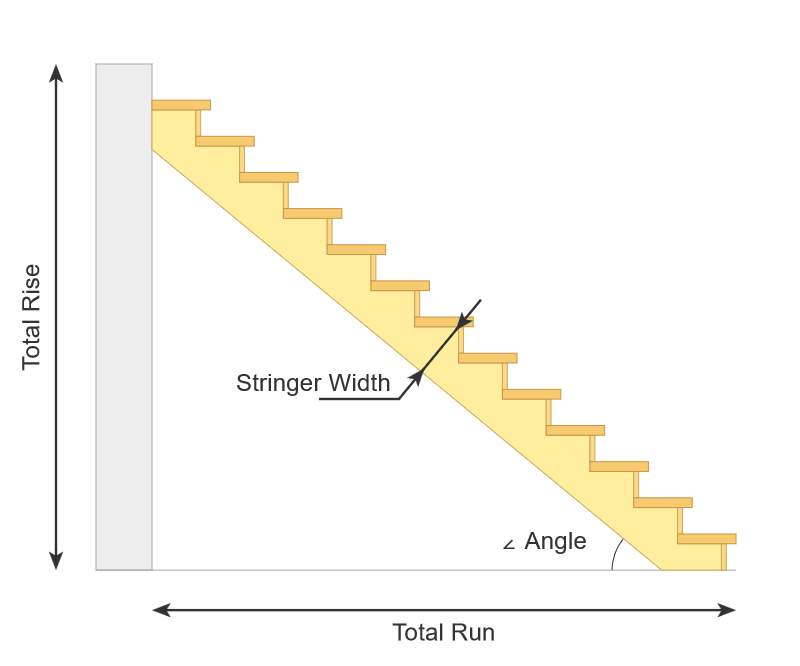Using these values, our calculator works out the vertical height rise of each individual step with the fraction:

$$Rise = {Total\,Rise \over Number\,of\,Steps}$$

In addition, our calculator allows you to choose between a standard and a flush mount.

Deciding to use one mount over the other will depend on your individual situation and DIY project.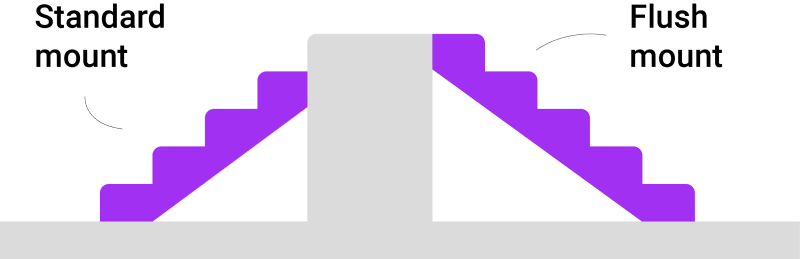Moving through the article and explanation these formulae are rather abstract and complicated.

This is intended to the calculations, formulae and explanations easier to follow.

## Example Calculations

• For both our examples below, we imagine we want to have 17 steps running up to a landing with a total vertical height rise of 120 inches and total horizontal run of 180 inches
• Let’s say that we have a tread thickness of 2 inches
• Tread overlap of 2 inches
• Riser thickness of 1 inch
• And a stringer width of 12 inches

We shall take you through the example calculations for first a standard mount, and then a flush mount set of stairs.

In doing so, we shall calculate the dimensions of the steps and the staircase.

In addition, we will show you how the stair stringer calculator works out the rise, run, angle and stringer length, allowing you to plan the layout of your intended staircase effectively.

Either, follow these calculations through, or simply use the calculator to get your DIY project off to a breeze!

Sound good? 😀

### Standard Mount Calculations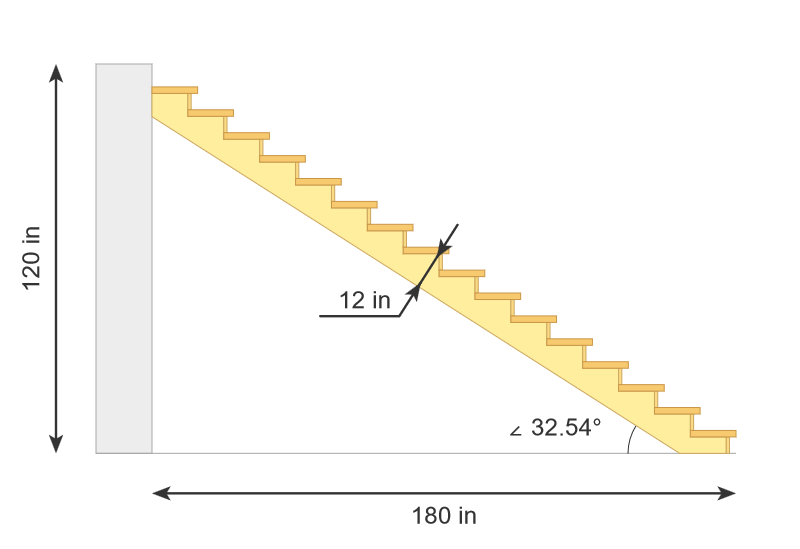First, we calculate the vertical height rise of each step:

$$Rise = {Total\,Rise \over Number\,of\,Steps} = {120\,in \over 17} = 7.06\,in$$

Now, using a standard mount staircase, the vertical height rise of the steps will be one step less than with a flush mount because the final step is taken up by the decking. Thus, we calculate:

$$Rise\,of\,steps = Total\,Rise – Rise = 120\,in – 7.06\,in = 112.94\,in$$

Now, if we include tread thickness (the thickness of the tread on each step), we must subtract this as well. The deck stair calculator thus works out:

$$Run\,of\,steps = Total\,Run – (Tread\,Overlap + Riser\,Thickness) = 180\,in – (2\,in + 1\,in) = 177\,in$$

Thus, our deck staircase calculator can figure out the total horizontal distance between the edge of each step (not forgetting that the final step is the decking!):

$$Run = {Run\,of\,steps \over Number\,of\,steps} = {177\,in \over 16} = 11.06\,in$$

Now, if we consider a single step of the staircase, the total horizontal distance will be given by:

$$Total\,Horizontal\,Distance = Run + Tread\,Overlap + Riser\,Thickness = 11.06\,in + 2\,in + 1\,in = 14.06\,in$$

And the angle (or pitch!) between the horizontal and the staircase is calculated by:

$$tan\,\alpha_1 = {Rise\,of\,steps \over Run\,of\,steps} → \alpha_1 = tan^{-1} {Rise\,of\,steps \over Run\,of\,steps} = tan^{-1} {112.94\,in \over 177\,in} = 32.54^o$$

Now, to find the stringer length of the staircase requires some more complicated Mathematics. 🧐

Keep following through the example below to see it in detail!

If we consider the stringer, the angle between the left-hand step and the horizontal (α2) is given by subtracting the previously calculated angle from 90 degrees: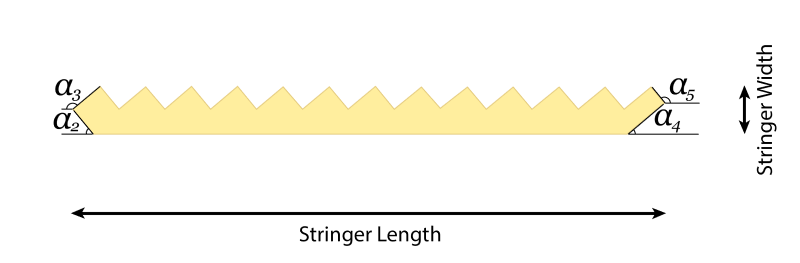$$\alpha_2 = 90^o – \alpha_1 = 90^o – 32.54^o = 57.46^o$$

Similarly,

$$\alpha_3 = 90^o + \alpha_2 = 90^o + 57.46^o = 147.46^o$$

And

$$\alpha_4 = \alpha_1 = 32.54^o$$

$$\alpha_5 = 90^o + \alpha_1 = 90^o + 32.54^o = 122.54^o$$

In addition, the calculator is able to figure out the horizontal and vertical dimensions of the stringer.

For ease of understanding, again, see the reference picture. The calculator works out all these distances using the method given below: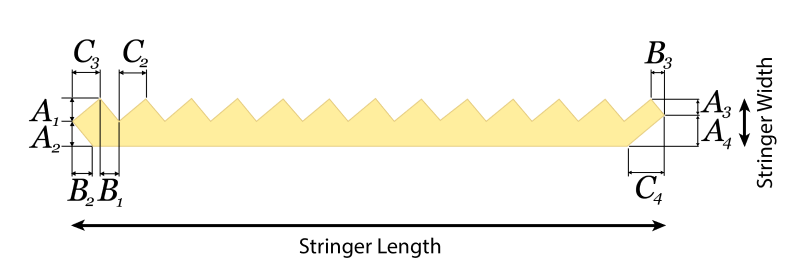$$A_1 = Rise × sin⁡\,\alpha_2 = 7.06\,in × sin\,57.46^o = 5.95\,in$$

$$A_2 = Stringer\,Width – A_1 = 12\,in – 5.95\,in = 6.05\,in$$

$$C_2 = \sqrt{Run^2 – {A_1}^2} = \sqrt{(11.06\,in)^2 – (5.95\,in)^2} = 9.32\,in$$

$$C_3 = A_1 × tan\,⁡\alpha_2 = 5.95\,in × tan\,57.46^o = 9.33\,in$$

$$B_1 = \sqrt{Rise^2 – {A_1}^2} = \sqrt{(7.06\,in)^2- (5.95\,in)^2} = 3.8\,in$$

$$B_2 = A_2 × tan⁡\,⁡\alpha_1 = 6.05\,in × tan⁡\,32.54^o = 3.86\,in$$

$$B_3 = (Rise -Tread\,Thickness) × cos⁡\,⁡\alpha_2 = (7.06\,in – 2\,in) × cos\,57.46^o = 2.72\,in$$

$$A_3 = (Rise – Tread⁡\,Thickness) × cos⁡⁡\,⁡\alpha_1 = (7.06⁡\,in – 2⁡\,in) × cos⁡⁡\,32.54^o = 4.26⁡\,in$$

$$A_4 = Stringer\,Width – A_3 = 12\,in – 4.27\,in = 7.74\,in$$

$$C_4 = A_4 × tan\,\alpha_2 = 7.74\,in × tan⁡\,57.46^o = 12.12\,in$$

Finally, the calculator combines the above calculated values to figure out the total stringer length of the steps:

$$Stringer\,Length = C_3 + B_3 + (Number\,of\,Steps – 1) × (B_1 + C_2) = 9.33\,in + 2.72\,in + (16-1) × (3.8\,in + 9.32\,in) = 208.88\,in$$

### Flush Mount Calculations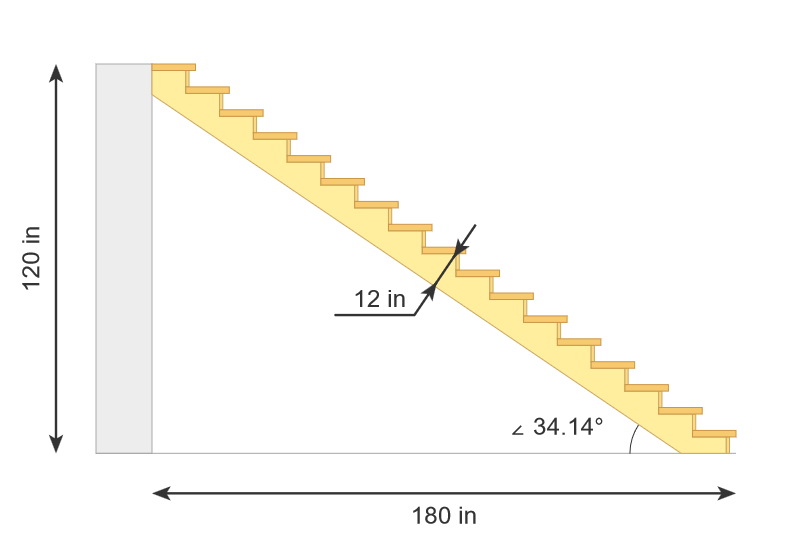Calculating the rise, run, angle and stringer length for a flush mount is very similar to the standard mount.

We simply remember that the final step takes us onto the decking.

As before, we calculate the vertical height rise:

$$Rise = {Total\,Rise \over Number\,of\,Steps} = {120\,in \over 17} = 7.06\,in$$

Unlike the standard mount measurement, where we subtracted the final step from the rise, with a flush mount we have that:

$$Rise\,of\,steps = Total\,Rise = 120\,in$$

However, on the top step, we will not have any tread (because the final step is the decking itself!) Thus, we transform the above formulae to get the vertical rise spacing:

$$Rise\,of\,steps = Total\,Rise – Tread\,Thickness = 120\,in – 2\,in = 118\,in$$

As before, including the tread when considering the run of the steps gives:

$$Run\,of\,steps = Total\,Run – (Tread\,Overlap + Riser\,Thickness) = 180\,in – (2\,in + 1\,in) = 177\,in$$

Thus, our calculator works out the total horizontal distance between the edge of each step.

The difference here is that we have 17 steps, as the final step is included in the flush mount!

$$Run = {Run\,of\,steps \over Number\,of\,steps} = {177\,in \over 17} = 10.41\,in$$

Consider a single step, the total horizontal distance will be given by:

$$Total\,Horizontal\,Distance = Run + Tread\,Overlap + Riser\,Thickness = 10.41\,in + 2\,in + 1\,in = 13.41\,in$$

And the angle between the horizontal and the stairs is once again given by:

$$tan\,\alpha_1 = {Rise\,of\,steps \over Run\,of\,steps} → \alpha_1 = tan^{-1} {Rise\,of\,steps \over Run\,of\,steps} = tan^{-1} {120\,in \over 177\,in} = 34.14^o$$

Calculating the stringer length is the same method as for the standard mount.

The calculator first figures out the angles:$$\alpha_2 = 90^o – \alpha_1 = 90^o – 34.14^o = 55.86^o$$

$$\alpha_3 = 90^o + \alpha_2 = 90^o + 55.86^o = 145.86^o$$

$$\alpha_4 = \alpha_1 = 34.14^o$$

$$\alpha_5 = 90^o + \alpha_1 = 90^o + 34.14^o = 124.14^o$$

And the calculator figures out the spacing for the lengths as before:$$A_1 = Rise × sin⁡\,\alpha_2 = 7.06\,in × sin\,55.86^o = 5.84\,in$$

$$A_2 = Stringer\,Width – A_1 = 12\,in – 5.84\,in = 6.16\,in$$

$$C_2 = \sqrt{Run^2 – {A_1}^2} = \sqrt{(10.41\,in)^2 – (5.84\,in)^2} = 8.62\,in$$

$$C_3 = A_1 × tan\,⁡\alpha_2 = 5.84\,in × tan\,55.86^o = 8.62\,in$$

$$B_1 = \sqrt{Rise^2 – {A_1}^2} = \sqrt{(7.06\,in)^2- (5.84\,in)^2} = 3.96\,in$$

$$B_2 = A_2 × tan⁡\,⁡\alpha_1 = 6.16\,in × tan⁡\,34.14^o = 4.17\,in$$

$$B_3 = (Rise -Tread\,Thickness) × cos⁡\,⁡\alpha_2 = (7.06\,in – 2\,in) × cos\,55.86^o = 2.84\,in$$

$$A_3 = (Rise – Tread⁡\,Thickness) × cos⁡⁡\,⁡\alpha_1 = (7.06⁡\,in – 2⁡\,in) × cos⁡⁡\,34.14^o = 4.19⁡\,in$$

$$A_4 = Stringer\,Width – A_3 = 12\,in – 4.19\,in = 7.81\,in$$

$$C_4 = A_4 × tan\,\alpha_2 = 7.81\,in × tan⁡\,55.86^o = 11.52\,in$$

Finally, the calculator works out the total stringer length as before:

$$Stringer\,Length = C_3 + B_3 + (Number\,of\,Steps – 1) × (B_1 + C_2) = 8.62\,in + 2.84\,in + (17-1) × (3.96\,in + 8.62\,in) = 212.72\,in$$

Be careful! ⚠️

Make sure you don’t round your answer before the final calculation. These small rounding errors can have a great difference on the final answer!

We understand that this is rather difficult to follow! Rest assured, if you don’t understand this, don’t worry.

The calculator does this all for you, shifting the emphasis and worry from you over to the technology. 😉

• What guidelines or regulations are there I need to consider when planning to build a set of stairs?

We recommend that you are aware of all building regulations in your home country.

Our calculator is programmed to give accurate measurements within the guidance of the Council of American Building Officials and International Code Council.

• Which properties must meet the building regulations of my home country for stairs and staircases?

You should check with the building regulations of your home country for an exact answer.

However, a good guide is that any business or church, organization, office or commercial property should meet the given stair standards of your home country.

Be careful – you open yourself up to potential substantial fines if you do not meet the stair standards.

Use our calculator, do some research and follow the above examples to make sure you are meeting them before getting started!

• What if my stair measurements are in other metric or imperial units?

The answer is very simple! Our calculator can work in many different units at the same time.

The estimator does the conversions for you using the following ratios:

$$1\,foot = 12\,inches = 0.33\,yards = 30.48\,centimeters = 0.3048\,meters$$

It’s that easy! 🙂

• Do my home stairs have to meet local governing standards?

No! As a general rule, building stair regulation standards are applicable for commercial properties using permanent stairs, not for typical home, temporary or portable steps for personal use.

• Is there any guidance or advice as to how long my stairs should be?

A good guideline is given by the Council of American Building Officials and International Code Council.

These regulations recommend the stair rise to be no more than 7.75 inches (17.78 cm) and stair run to be no less than 10 inches (25.4 cm).

Don’t forget though, this is for a commercial property.

This may not be practical or necessary for your home, where your stairs do not have to meet the same commercial standards, but they should be used as a guide.Add live graphics
Copied to clipboard! Preview